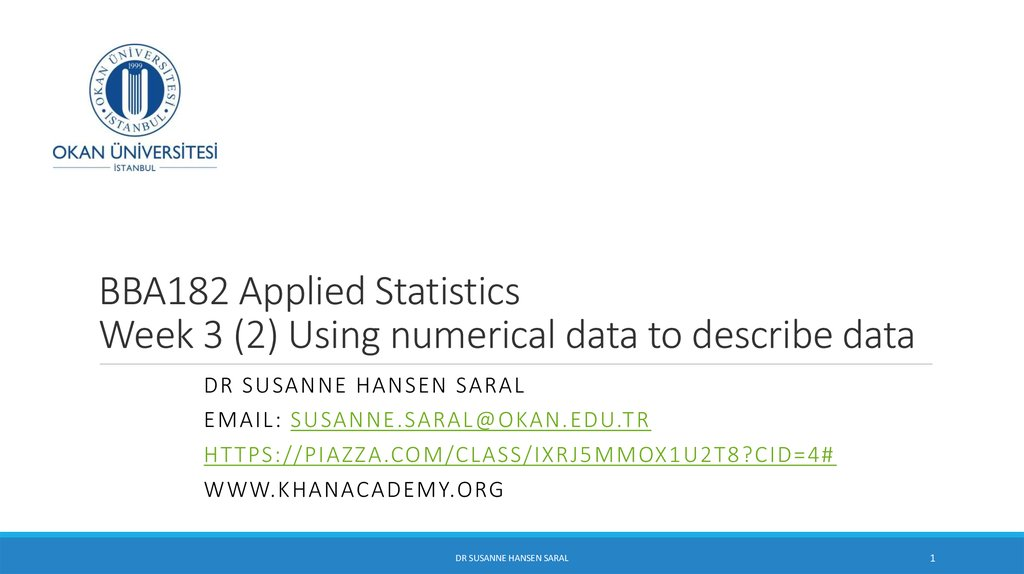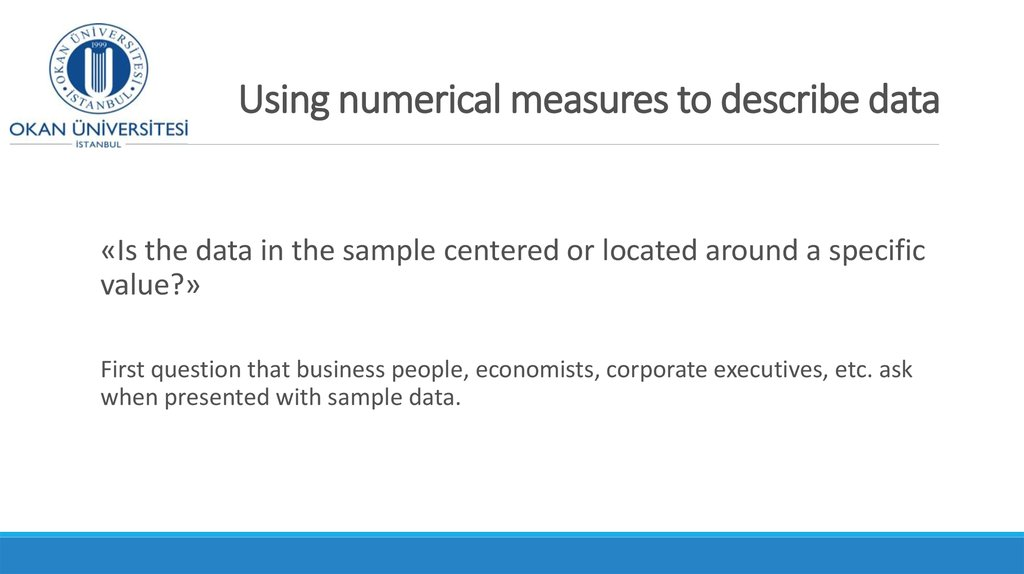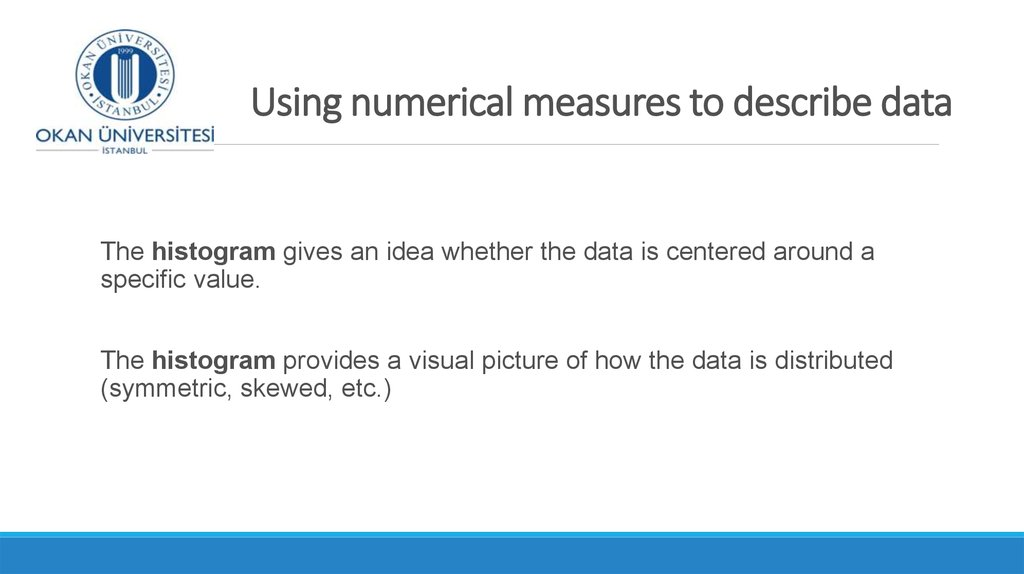# Using numerical measures to describe data. Measures of the center. Week 3 (2)

## 1. BBA182 Applied Statistics Week 3 (2) Using numerical data to describe data

DR SUSANNE HANSEN SARAL
EMAIL: [email protected]
HT TPS://PIAZZA.COM/CLASS/IXRJ5MMOX1U2T8?CID=4#
DR SUSANNE HANSEN SARAL
1

## 2. Using numerical measures to describe data

«Is the data in the sample centered or located around a specific
value?»
when presented with sample data.

## 3. Using numerical measures to describe data

The histogram gives an idea whether the data is centered around a
specific value.
The histogram provides a visual picture of how the data is distributed
(symmetric, skewed, etc.)

## 5. Numerical measures to describe data

Describing Data Numerically
Central Tendency
Variation
Mean
Range
Median
Interquartile Range
Mode
Variance
Standard Deviation
Coefficient of Variation
Ch. 2-5

## 6. Measures of the center of the data set

2.1
Measures of the center of the data set
Measures of Central
Tendency
Mean
Median
Mode
n
x
x
i 1
i
n
Arithmetic
average of the
data
Midpoint of
ranked/ordered
values in the data
Most frequently
observed value in the
data
(if one exists)